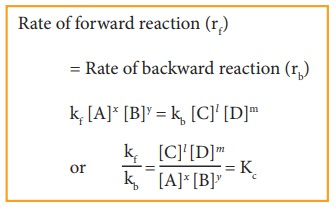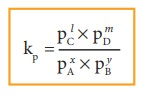Home | | Chemistry 11th std | Equilibrium constants (Kp and Kc)

# Equilibrium constants (Kp and Kc)

Chemistry : Physical and Chemical Equilibrium

Equilibrium constants (Kp and KC):

Let us consider a reversible reaction,

xA+yB  lC + mDwhere, A and B are the reactants, C and D are the products and x,y, l and m are the stoichiometric coefficients of A, B, C and D, respectively.

Applying the law of mass action, the rate of the forward reaction,

rf α [A]x [B]y (or) rf = kf [A]x [B]y

Similarly, the rate of the backward reaction,

rb α [C]l [D]m

(or)

rb = kb [C]l[D]m

where kf and kb are proportionality constants

At equilibrium,

Rate of forward reaction (rf) = Rate of backward reaction (rb)

kf [A]x [B]y = kb [C]l [D]mwhere, Kc is the equilibrium constant in terms of concentration (active mass).

At a given temperature, the ratio of the product of active masses of reaction products raised to the respective stoichiometric coefficients in the balanced chemical equation to that of the reactants is a constant, known as equilibrium constant. Later when we study chemical kinetics we will learn that this is only approximately true.

If the reactants and products of the above reaction are in gas phase, then the equilibrium constant can be written in terms of partial pressures as indicated below,Where, pA, pB, pC,and pD are the partial pressures of the gas A, B, C and D, respectively.

Study Material, Lecturing Notes, Assignment, Reference, Wiki description explanation, brief detail
11th Chemistry : UNIT 8 : Physical and Chemical Equilibrium : Equilibrium constants (Kp and Kc) |